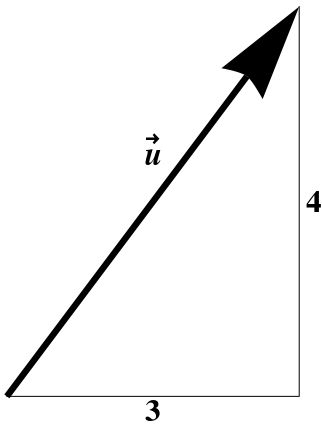## Section1.6Unit Vectors

Displacement vectors have a length, which you can measure with a ruler. The corresponding notion for velocity vectors is the speed at which you are going. In either case, you can measure the angle between two such vectors using a protractor.

We use the terminology vectors in space, or vectors in the plane to refer to such geometric vectors, which have both a magnitude (in appropropriate units) and a direction. We will assume for now that all vectors are of this type, that is, that they have both direction and magnitude.  1

The magnitude of $$\ww$$ is denoted by $$|\ww|\text{,}$$ also written $$||\ww||$$ or sometimes just as $$w\text{,}$$ without an arrow on top. The magnitude of $$\ww$$ is often casually called the “length” of $$\ww\text{,}$$ but that usage is only correct if $$\ww$$ has dimensions of length. The displacement between two points does indeed have a length, but to talk about your speed as being the “length” of your velocity vector is confusing at best.

For example, suppose you are moving at 5 miles per hour (mph) in a roughly northeasterly direction. Your velocity $$\ww$$ could be represented as shown in Figure 1.4.1. The magnitude of $$\ww$$ would be 5 mph, and its direction could be specified as an angle from due north.

A unit vector is a vector whose magnitude is $$1\text{.}$$ Given any vector $$\ww\text{,}$$ we get a unit vector that points in the same direction as $$\ww$$ by dividing $$\ww$$ by its magnitude $$|\ww|\text{,}$$ that is, $$\ww\over|\ww|$$ is a unit vector.

Unit vectors are dimensionless!

If you divide your velocity by your speed, both measured in miles per hour, the magnitude of the result is just plain $$1\text{,}$$ not$$1$$ mph”. What information is left? The direction you are going. We therefore define the direction of a vector $$\ww$$ to be the unit vector in the same direction, which is just $$\ww\over|\ww|\text{.}$$

As is customary in many branches of science, we will denote unit vectors with a hat, rather than an arrow. We will write the unit vectors in the $$x\text{,}$$ $$y\text{,}$$ and $$z$$ directions as $$\xhat\text{,}$$ $$\yhat\text{,}$$ and $$\zhat\text{,}$$ respectively. There are other common notations for these vectors. For instance, you may see $$\xhat$$ written instead as any of $$\iv\text{,}$$ $$\ii\text{,}$$ $$\aa_x\text{,}$$ or $$\ee_x\text{.}$$ You may also see the notation $$w$$ and $$\Hat{w}$$ for the magnitude and direction, respectively, of a given vector $$\ww\text{,}$$ so that $$w=|\ww|$$ and $$\Hat{w}={\ww\over |\ww|}\text{.}$$

Any vector $$\ww$$ in three dimensions can of course be expressed in terms of its components in the $$x\text{,}$$ $$y\text{,}$$ and $$z$$ directions. For instance, we might have $$\ww = 2\,\xhat + 3\,\yhat + 4\,\zhat\text{.}$$ But it is important to think of vectors as arrows in space, rather than as a list of three components. While these points of view are equivalent, the former is more geometric. One of the goals of this book is to develop this geometric intuition so that it can be used in applications. It is in part for this reason that, unlike many texts, we almost always include the basis vectors $$\{\xhat,\yhat,\zhat\}$$ when referring to the (Cartesian) components of a vector; we avoid writing “$$\ww=\langle 2,3,4\rangle$$”. Thus, for an arbitrary vector $$\ww$$ with unknown (Cartesian) components, we write

\begin{equation} \ww = w_x\,\xhat + w_y\,\yhat + w_z\,\zhat\tag{1.6.1} \end{equation}

### Notation.

Note the use of subscripts to denote the components; these subscripts do not denote partial differentiation, and in fact for this reason we never use subscripts to denote derivatives.Figure 1.6.1. The vector $$\uu\text{,}$$ showing its (Cartesian) components.

For example, the components of the vector $$\uu$$ above in Cartesian coordinates are shown in Figure 1.6.1. If $$\uu$$ refers to your velocity, this example corresponds to your moving at 3 mph to the east and 4 mph to the north, for an overall velocity of 5 mph in the direction

\begin{equation*} \frac{\uu}{|\uu|} = \frac35\xhat + \frac45\yhat \end{equation*}

(which could also have been specified as a compass direction).

Not all vectors have magnitudes!